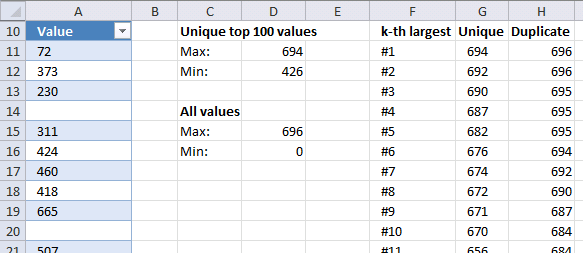Author: Oscar Cronquist Article last updated on October 31, 2019This article explains how to calculate the largest and smallest number based on a condition which is if the number is unique. Unique meaning it exists only once in a column, numbers that have duplicates are not calculated.

I will also demonstrate how to calculate the k-th smallest or largest value based on the same condition using an array formula.

The image above shows a formula in cell D11, it extracts the largest unique number from column A. Cell D12 contains a formula that extracts the 100th largest unique number from column A.

How to find Min and Max numeric values in a range of cells that have duplicate numbers and blanks, but only want to find the Min and Max on the largest/top 100 non-duplicate values.

• Build formulas that extract the min and max number if the number itself is unique.
• Identify unique numbers in a column.
• Create a formula that returns the k-th largest and smallest number if unique

Array formula in cell D11:

=MAX(IF((COUNTIF(Table1[Value],Table1[Value])< > 1)+(Table1[Value]=""),"",Table1[Value]))

To enter an array formula press and hold CTRL + SHIFT simultaneously, then press Enter once. Release all keys.

The formula bar now shows the formula enclosed with curly brackets telling you that you entered the formula successfully. Don't enter the curly brackets yourself.

You will find an explanation of this formula below.

Array formula in cell D12:

=LARGE(IF((COUNTIF(Table1[Value],Table1[Value])< > 1)+(Table1[Value]=""),"",Table1[Value]),100)

This array formula returns the 100th largest unqiue number from column A.

Formula in cell D15:

=MAX(Table1[Value])

This formula returns the largest number from column A, note it will also return a number that has a duplicate.

Formula in cell D16:

=MIN(Table1[Value])

This formula returns the smallest number from column A, note it will also return a number that has a duplicate.

Array formula in cell G11:

=LARGE(IF((COUNTIF(Table1[Value],Table1[Value])< > 1)+(Table1[Value]=""),"",Table1[Value]),ROW(A1))

This array formula is dynamic meaning it returns a different value in each cell, simply copy cell G11 and paste it to cells below. The ROW function utilizes a relative cell reference that changes when the formula is copied.

### Explaining array formula in cell D11

#### Step 1 - Count the number of cells within a range that meet a given condition

The COUNTIF function counts cells that equals a specific value, the formula performs multiple calculations if we use multiple values and enter the formula as an array formula.

Note, we are using the same values in the first argument as in the second argument. The COUNTIF function returns a number larger than 1 if a value has a duplicate. COUNTIF(rangecriteria)

COUNTIF(Table1[Value],Table1[Value])

returns

{1; 1; 1; 0; 1; 1; 1; 1; 1; ...}.

#### Step 2 - Check if value in array is a duplicate

The less than and larger than characters allow you to create a logical expression that returns either TRUE or FALSE.

COUNTIF(Table1[Value],Table1[Value])<> 1

becomes

{1; 1; 1; 0; 1; 1; 1; 1; 1; ...}<> 1

and returns

{FALSE; FALSE; FALSE; TRUE; FALSE; FALSE; FALSE; FALSE; FALSE; ...}.

#### Step 3 - Check if table value is a blank

We also need to figure out how to ignore blanks in column A, the equal sign lets you compare each value with nothing. It returns TRUE if a cell is blank.

(Table1[Value]="")

returns

{FALSE; FALSE; FALSE; TRUE; FALSE; FALSE; FALSE; FALSE; FALSE; ...}.

#### Step 4 - Return table value if conditions are met

The IF function lets your perform different actions based on a logical expression, in this case we want the formula to return "" (nothing) if TRUE and return the number itself if false.

IF((COUNTIF(Table1[Value],Table1[Value])< > 1)+(Table1[Value]=""),"",Table1[Value])

becomes

IF({FALSE; FALSE; FALSE; TRUE; FALSE; FALSE; FALSE; FALSE; FALSE; ...}, "", {72; 373; 230; 0; 311; 424; 460; 418; 665})

and returns

{72; 373; 230; ""; 311; 424; 460; 418; 665; ...}.

#### Step 5 - Return max value

The MAX function simply returns the largest number in the array or cell range.

MAX(IF((COUNTIF(Table1[Value],Table1[Value])< > 1)+(Table1[Value]=""),"",Table1[Value]))

becomes

=MAX({72; 373; 230; ""; 311; 424; 460; 418; 665; ...})

and returns 694 in cell D11.

### Get the Excel fileFind-max-unique-value-from-a-range-that-have-duplicate-numbers-and-blanks.xlsx